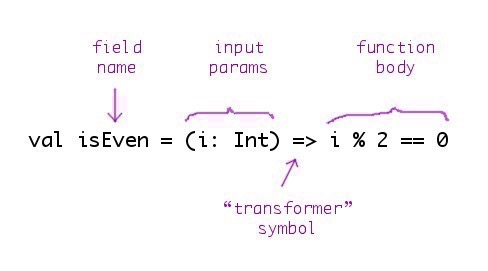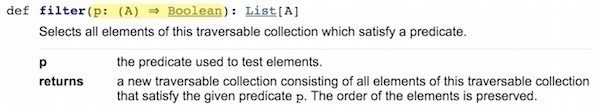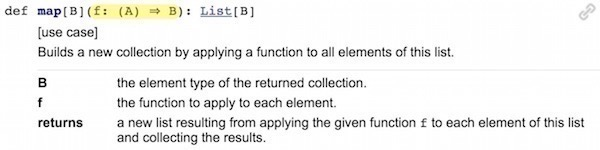# Functions Are Variables, Too

This is a page from my book, Functional Programming, Simplified

“A variable is a named entity that refers to an object. A variable is either a `val` or a `var`. Both vals and vars must be initialized when defined, but only vars can be later reassigned to refer to a different object.”

The Scala Glossary

## Goals

The goal of this lesson is to show that in a good FP language like Scala, you can use functions as values. In the same way that you create and use `String` and `Int` values, you can use a function:

``````val name = "Al"                    // string value
val weight = 222                   // int value
val double = (i: Int) => i * 2     // function value``````

To support this goal, this lesson shows:

• How to define a function as a `val`
• The “implicit” form of the `val` function syntax
• How to pass a function to another function
• Other ways to treat functions as values

## Scala’s `val` function syntax

Understanding Scala’s `val` function syntax is important because you’ll see function signatures over and over in a variety of places, including:

• When you define `val` functions
• When you define function input parameters (i.e., when one function takes another function as an input parameter)
• When you’re reading the Scaladoc for almost every method in the Scala collections classes
• In the REPL output

You’ll see examples of most of these in this lesson.

## Formalizing some definitions

Before getting into this lesson, it will help to make sure that I’m formal about how I use certain terminology. For instance, given this expression:

``val x = 42``

it’s important to be clear about these things:

1. Technically, `x` is a variable, a specific type of variable known as an immutable variable. Informally, I prefer to refer to `x` as a “value,” as in saying, “`x` is an integer value.” I prefer this because `x` is declared as a `val` field; it’s bound to the `Int` value 42, and that can never change. But to be consistent with (a) other programming resources as well as (b) algebraic terminology, I’ll refer to `x` as a variable in this lesson.

Wikipedia states that in algebra, “a variable is an alphabetic character representing a number, called the value of the variable, which is either arbitrary or not fully specified or unknown.” So in this way, refering to `x` as a variable is consistent with algebraic terms.

1. `x` has a type. In this case the type isn’t shown explicitly, but we know that the type is an `Int`. I could have also defined it like this:

val x: Int = 42

But because programmers and the Scala compiler know that `42` is an `Int`, it’s convenient to use the shorter form.

1. Variables themselves have values, and in this example the variable `x` has the value `42`. (As you can imagine, it might be confusing if I wrote, “The value `x` has the value `42`.)

I’m formalizing these definitions now because as you’re about to see, these terms also apply to creating functions: functions also have variable names, types, and values.

## Function literals

If you haven’t heard of the term “function literal” before, it’s important to know that in this example:

``xs.map(x => x * 2)``

this part of the code is a function literal:

``x => x * 2``

It’s just like saying that this is a string literal:

``"hello, world"``

I mention this because ...

## Function literals can be assigned to variables

In functional programming languages, function literals can be assigned to variable names. In Scala this means:

• You can define a function literal and assign it to a `val` field, which creates an immutable variable
• You give that variable a name, just like any other `val` field
• A function variable has a value, which is the code in its function body
• A function variable has a type — more on this shortly
• You can pass a function around to other functions, just like any other `val`
• You can store a function in a collection, such as a `Map`
• In general, you use a function variable just like any other variable

## The `val` function syntax

In the “Explaining the `val` Function Syntax” appendix, I show two different ways to define functions using `val`s in Scala. In this lesson I’ll use only the following approach, which shows the “implicit return type” syntax:

``val isEven = (i: Int) => i % 2 == 0``

In this case “implicit” means that this function doesn’t explicitly state that it returns a `Boolean` value; both you and the compiler can infer that by looking at the function body.

Scala also has a `val` function syntax where you explicitly declare the function’s return type, and I show that in the appendix.

I discuss the implicit syntax in detail in the appendix, but here’s a quick look at what each of those fields means:If that syntax looks a little unusual, fear not, I show more examples of it in this lesson and in the appendices.

### Other ways to write this function

This function body is a short way of saying that it returns `true` if the `Int` it is given is an even number, otherwise it returns `false`. If you don’t like the way that code reads, it may help to put curly braces around the function body:

``val isEven = (i: Int) => { i % 2 == 0 }``

Or you can make the if/else condition more obvious:

``val isEven = (i: Int) => if (i % 2 == 0) true else false``

You can also put curly braces around that function body:

``val isEven = (i: Int) => { if (i % 2 == 0) true else false }``

Finally, if you prefer a really long form, you can write `isEven` like this:

``````val isEven = (i: Int) => {
if (i % 2 == 0) {
true
} else {
false
}
}``````

Note: I only show this last version to show an example of a multi-line function body. I don’t recommend writing short functions like this.

If you were going to explain any of these functions to another person, a good explanation would be like this:

“The function `isEven` transforms the input `Int` into a `Boolean` value based on its algorithm, which in this case is `i % 2 == 0`.

## The general implicit `val` function syntax

You can come to understand the implicit `val` function syntax by pasting a few functions into the Scala REPL. For instance, when you paste this function into the REPL:

``val isEven = (i: Int) => i % 2 == 0``

you’ll see that the REPL responds by showing that `isEven` is an instance of something called `<function1>`:

``````scala> val isEven = (i: Int) => i % 2 == 0
isEven: Int => Boolean = <function1>``````

And when you paste a function that takes two input parameters into the REPL:

``val sum = (a: Int, b: Int) => a + b``

you’ll see that it’s an instance of `<function2>`:

``````scala> val sum = (a: Int, b: Int) => a + b
sum: (Int, Int) => Int = <function2>``````

When I line up the REPL output for those two examples, like this:

``````isEven:  Int        => Boolean  =  <function1>
sum:     (Int, Int) => Int      =  <function2>``````

you can begin to see that the general form for the way the REPL displays function variables is this:

``variableName: type = value``

You can see this more clearly when I highlight the function types and values. This is the REPL output for `isEven`:

``````isEven: Int => Boolean = <function1>
------  --------------   -----------
name         type           value``````

and this is the output for the `sum` function:

``````sum:   (Int, Int) => Int  =  <function2>
----   -----------------     -----------
name         type               value``````

The type of the `isEven` function can be read as, “Transforms an `Int` value into a `Boolean` value,” and the `sum` function can be read as, “Takes two `Int` input parameters and transforms them into an `Int`.”

Cool FP developers generally don’t say, “a function returns a result.” They say things like, “a function transforms its inputs into an output value.” Or, as it’s stated in the Land of Lisp book, Lisp purists prefer to say that “a function evaluates to a result.” This may seem like a minor point, but I find that using phrases like this helps my brain to think of my code as being a combination of algebraic functions (or equations) — and that’s a good way to think.

## What `<function1>` and `<function2>` mean

In the “Explaining the `val` Function Syntax” appendix I write more about this topic, but in short, the output `<function1>` indicates that `isEven` is an instance of the `Function1` trait (meaning that it has one input parameter), and `<function2>` means that `sum` is an instance of the `Function2` trait (meaning that it has two input parameters). The actual “value” of a function is the full body of the function, but rather than show all of that, the REPL uses `<function1>` and `<function2>` to show that `isEven` and `sum` are instances of these types.

As I discuss in that appendix, behind the scenes the Scala compiler converts this function:

``val sum = (a: Int, b: Int) => a + b``

into code that looks a lot like this:

``````val sum = new Function2[Int, Int, Int] {
def apply(a: Int, b: Int): Int = a + b
}``````

I don’t want to get too hung up on these details right now, but this is where the `Function2` reference comes from. For more information on this topic, see the “Explaining the `val` Function Syntax” appendix.

## Passing functions into other functions

A great thing about functional programming is that you can pass functions around just like other variables, and the most obvious thing this means is that you can pass one function into another. A good way to demonstrate this is with the methods in the Scala collections classes.

For example, given this list of integers (`List[Int]`):

``val ints = List(1,2,3,4)``

and these two functions that take `Int` parameters:

``````val isEven = (i: Int) => i % 2 == 0
val double = (i: Int) => i * 2``````

you can see that `isEven` works great with the `List` class `filter` method:

``````scala> ints.filter(isEven)
res0: List[Int] = List(2, 4)``````

and the `double` function works great with the `map` method:

``````scala> ints.map(double)
res1: List[Int] = List(2, 4, 6, 8)``````

Passing functions into other functions like this is what functional programming is all about.

### How this works (the short answer)

In the upcoming lessons on Higher-Order Functions I show how to write methods like `map` and `filter`, but here’s a short discussion of how the process of passing one function into another function (or method) works.

Technically `filter` is written as a method that takes a function as an input parameter. Any function it accepts must (a) take an element of the type contained in the collection, and (b) return a `Boolean` value. Because in this example `filter` is invoked on `ints` — which is a `List[Int]` — it expects a function that takes an `Int` and returns a `Boolean`. Because `isEven` transforms an `Int` to a `Boolean`, it works great with `filter` for this collection.

### A look at the Scaladoc

The `filter` method Scaladoc is shown in the next image. Notice how it takes a predicate which has the generic type `A` as its input parameter, and it returns a `List` of the same generic type `A`. It’s defined this way because `filter` doesn’t transform the list elements, it just filters out the ones you don’t want.As shown in the next image, `map` also takes a function that works with generic types. In my example, because `ints` is a `List[Int]`, you can think of the generic type `A` in the image as an `Int`. Because `map` is intended to let you transform data, the generic type `B` can be any type. In my example, `double` is a function that takes an `Int` and returns an `Int`, so it works great with `map`.I explain this in more detail in upcoming lessons, but the important point for this lesson is that you can pass a function variable into another function.

## Because functions are variables ...

Because functions are variables, you can do all sorts of things with them. For instance, if you define two functions like this:

``````val double = (i: Int) => i * 2
val triple = (i: Int) => i * 3``````

you can have fun and store them in a `Map`:

``````val functions = Map(
"2x" -> double,
"3x" -> triple
)``````

If you put that code into the REPL, you’ll have two functions stored as values inside a `Map`.

Now that they’re in there, you can pass the `Map` around as desired, and then later on get references to the functions using the usual `Map` approach, i.e., by supplying their key values. For example, this is how you get a reference to the `double` function that’s stored in the `Map`:

``````scala> val dub = functions("2x")
d: Int => Int = <function1>``````

This is just like getting a `String` or an `Int` or any other reference out of a `Map` — you specify the key that corresponds to the value.

Now that you have a reference to the original `double` function, you can invoke it:

``````scala> dub(2)
res0: Int = 4``````

You can do the same things with the other function I put in the `Map`:

``````scala> val trip = functions("3x")
t: Int => Int = <function1>

scala> trip(2)
res1: Int = 6``````

These examples show how to create functions as variables, store them in a `Map`, get them out of the `Map`, and then use them.

### The point of this example

Besides showing how to put function variables into `Map`s, a key point of this example is: in Scala you can use a function variable just like a `String` variable or an `Int` variable. The sooner you begin treating functions as variables in your own code, the further you’ll be down the path of becoming a great functional programmer.

### Exercise

Given what I’ve shown so far, this request may be a bit of an advanced exercise, but ... here’s that `Map` example again:

``````val functions = Map(
"2x" -> double,
"3x" -> triple
)``````

Given that `Map`, sketch its data type here:

``````|
|
|``````

As an example of what I’m looking for, this `Map`:

``val m = Map("age" -> 42)``

has a data type of:

``Map[String, Int]``

That’s what I’m looking for in this exercise: the type of the `Map` named `functions`.

### Solution to the exercise

If you pasted the `Map` code into the REPL, you saw its output:

``Map[String, Int => Int] = Map(2x -> <function1>, 3x -> <function1>)``

The first part of that output shows the `Map`’s data type:

``Map[String, Int => Int]``

The data type for the `Map`’s key is `String`, and the type for its value is shown as `Int => Int`. That’s how you write the type for a function that transforms a single `Int` input parameter to a resulting `Int` value. As you know from the previous discussion, this means that it’s an instance of the `Function1` trait.

As a second example, if the `Map` was holding a function that took two `Int`’s as input parameters and returns an `Int` — such as the earlier `sum` function — its type would be shown like this:

``Map[(Int, Int) => Int]``

That would be a `Function2` instance, because it takes two input parameters.

## Examples of `val` functions

To help you get comfortable with the “implicit return type” version of the `val` function syntax, here are the functions I showed in this lesson:

``````val isEven = (i: Int) => i % 2 == 0
val sum = (a: Int, b: Int) => a + b
val double = (i: Int) => i * 2
val triple = (i: Int) => i * 3``````

And here are a few more functions that show different input parameter types:

``````val strlen = (s: String) => s.length
val concat = (a: String, b: String) => a + b

case class Person(firstName: String, lastName: String)
val fullName = (p: Person) => s"\${p.firstName} \${p.lastName}"``````

## Summary

Here’s a summary of what I showed in this lesson:

• Function literals can be assigned to `val` fields to create function variables
• To be consistent with algebra and other FP resources, I refer to these fields are variables rather than values
• Examples of the `val` function syntax
• A function is an instance of a `FunctionN` trait, such as `Function1` or `Function2`
• What various function type signatures look like in the REPL
• How to pass a function into another function
• How to treat a function as a variable by putting it in a `Map`
• That, in general, you can use a function variable just like any other variable

In regards to `val` function signatures, understanding them is important because you’ll see them in many places, including function literals, the Scaladoc, REPL output, and other developer’s code. You’ll also need to know this syntax so you can write your own functions that take other functions as input parameters.

## What’s next

The next lesson shows that you can use `def` methods just like `val` functions. That’s important because most developers prefer to use the `def` method syntax to define their algorithms.

• Appendix: Explaining the `val` Function Syntax (TODO)
• Appendix: The Differences Between `val` and `def` When Creating Functions (TODO)